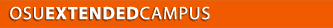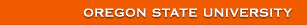Welcome Contact Getting Started Site Map Project 1 2 3 4 5 6 7 8 9 10 11

# Topic 3 - Review of Fundamentals

## Atomic And Nuclear Structure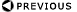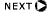### Outline

• Atomic Structure
• Rutherfords Atom
• Bohrs Model
• Modification of Bohrs Model
• Periodic Table
• Characteristic X-rays
• Nuclear Structure
• Isotopes / Nuclides
• Binding Energy
• Nuclear Models
• Nuclear Stability

### Rutherfords Nuclear Atom

• 1911
• Postulated positive charge in center of atom, called NUCLEUS
• Hypothesis tested by Geiger and Marsden
• Gold foil experiment
• Alpha particles
• Experimental result
• R = 1.2 x 10-15A1/3 m

### Rutherfords Atom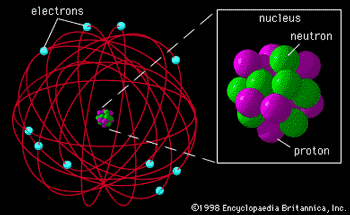### Gold Foil Experiment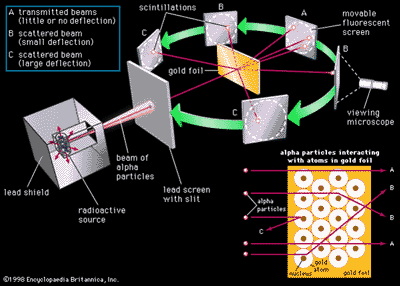### Bohrs Atomic Model

• Predecessor model: solar system concept
• Issues: classical theory predicted model unstable
• Resolution: 1913, Neils Bohr  denied validity of classical electromagnetic theory
• New: (Max Planck) quantum theory of radiation

### Bohrs Model for Hydrogen

• Explained observed spectrum
• Assumed for electron:
• travels around nucleus in a circular orbit
• energy in an orbit is proportional to its distance from the nucleus. The further from the nucleus, the more energy it has
• if it stays in a stationary orbit, it does not emit radiation
• Only a limited number of orbits with certain energies are allowed (quantization of orbits using Planck's hypothesis)
• orbits have angular momentum as integral multiple of Planck's constant divided by 2<p
• can only pass from one stationary state to another.
• Radiation is absorbed when one jumps to a higher energy orbit
• emitted when an one falls into a lower energy orbit.
• The energy of the radiation emitted or absorbed is exactly equal to the difference between the energies of the orbits

### Bohr Atomic Model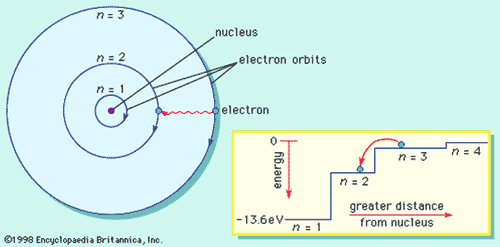### Modification of the Bohr Atom

• Bohr theory inadequate for even H atom
• Splitting of spectral lines
• Sublevels of energy
• Orbits are elliptical, not spherical, restricted by quantum conditions
• Describing atom by 4 quantum numbers

### Quantum Numbers

• A>ny of several quantities that identify the state of a physical system such as an atom, a nucleus, or a subatomic particle
• he principal quantum number for atomic electrons indicates the energy state and the probability of finding the electrons at various distances from the nucleus
• The larger this number, the greater the energy is and the farther the electron is likely to be from the nucleus
• The four quantum number are sufficient to uniquely characterize uniquely each atomic electron
 Symbol Quantum Number Value n Principal 1,2,……… l Azimuthal 0 to n -1 m Magnetic -l to +l s Spin -1/2, + 1/2
• Three quantum numbers are needed to specify each orbital in an atom
• the most important is the principal quantum number, n (the same that Bohr introduced)
• The principal quantum number specifies the energy of the electron in the orbital
• As n increases from its lowest value 1 through its allowed values 2, 3, . . . , the energies of the corresponding orbitals increase.

### Principle Quantum Number

• n labels the shell of the atom.
• Each shell
• has n2 individual orbitals with the same principal quantum number
• has orbitals that lie at approximately the same distance from the nucleus.
• esembles the layers of an onion, with successive shells surrounding the inner shells.

### Principal Quantum Number

• Hydrogen ground state
• n = 1
• 1 electron in the orbital closest to the nucleus.
• Ionization energy
• energy required to elevate the electron from   n = 1 to n = ¥
• energy required to remove the electron completely from the atom.

### Azimuthal Quantum Number

• orbital angular momentum quantum number.
• It represents the magnitude of the orbital angular momentum of the electron around the nucleus.
• as l increases, the rate at which the electron circulates around the nucleus increases.
• The values of l in a shell of principal quantum number n are limited to the n
values 0, 1, 2, . . . , n - 1
• the value of l in a given shell determines the subshell to which that orbital belongs.
• n subshells in a shell of principal quantum number n.
• 2l + 1 orbitals in a given subshell.
• conventional labels for subshells:
• l = 0 is called an s subshell,
• l = 1 is called a p subshell,
• l = 2 is called a d subshell.
• Other subshells
• The three subshells of the shell with n = 3 are called the 3s, 3p, and 3 d

### Magnetic Quantum Number

• Subshell l consists of 2 l + 1 individual orbitals.
• s subshell (l = 0) consists of a single s orbital
• p subshell (l = 1) consists of three p orbitals;
• d subshell (l = 2) consists of five d orbitals
• The individual orbitals are labeled with the magnetic quantum number, ml, which can take the 2 l + 1 values l, l - 1, . . . , -l.

### Quantum Recap

• n (principal quantum number) defines electron shells. Values are integers (n = 1,2,3,4,...).
• l (azimuthal quantum number)defines atomic orbitals. Values are integers from 0 to n-1.
• m (magnetic quantum number) defines number of orbitals of a given kind. Values are integers ranging from -l through 0 to +l.
• s (spin quantum number) has values of +½ and -½ (spin up, spin down)
• From Pauli Exclusion Principle: no two electrons can have the same set of four quantum numbers

### s orbital example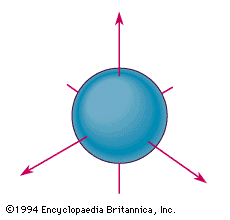The spherical boundary surface of an s orbital. This sphere shows the region of space in which there is the highest probability of finding an electron that is described by the corresponding wavefunction

### p orbitals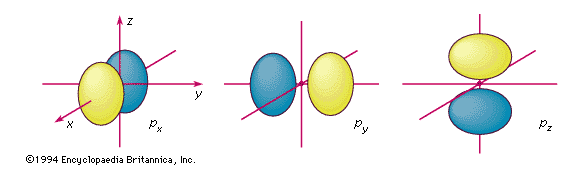The boundary surfaces of the three p orbitals of a given shell. They are labeled according to their orientation relative to the three axes. An electron described by one of these wavefunctions will not be found at the nucleus; there is a nodal plane running through the nucleus between the two lobes.

### d orbitals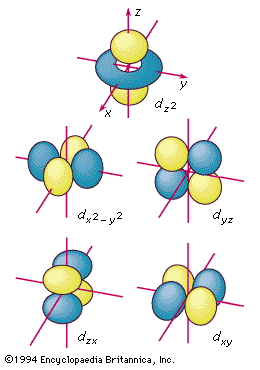The boundary surfaces of the five d orbitals of a given shell, appropriately labeled.

### Periodic Table of the Elements

• Constructed with Bohrs atomic model by application of Pauli exclusion principle

### Periodic Table of the Elements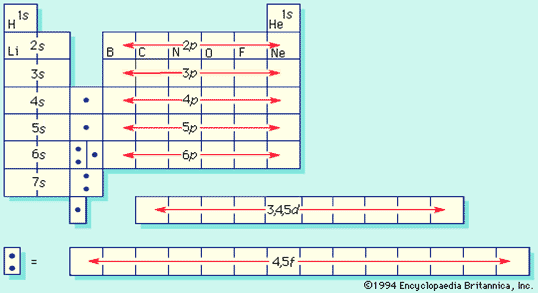### Periodic Table, again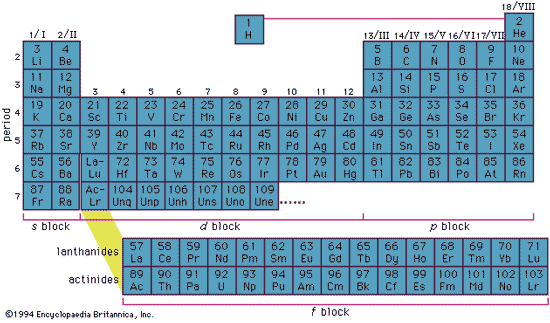### Electronic Structure

• Inner complete electron shells constitute the core or kernel
• Binding energy of the kernel electrons is much higher than that of the valence or conduction electrons.
• Kernel electrons remain practically undisturbed in most of the processes in which the atom participates.

### Consequence of Periodicity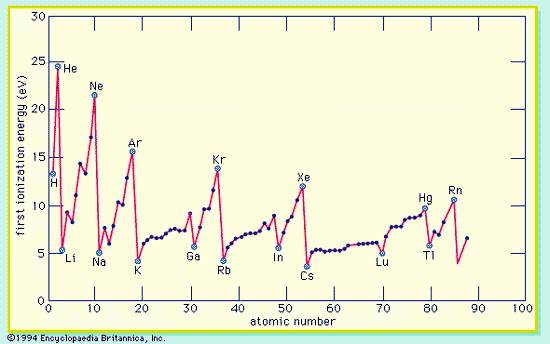### Characteristic X-rays

• Electron ejected from the kernel (e.g, K shell)
• States with n=1,2,3,4 constitute the K,L,M,M,.. shells
• Empty state (or hole) is left in K-shell
• Another electron in a higher energy level can fall into the vacant state
• Radiation emitted by electron "falling" into  vacant state is in the X-ray spectral region
• Electron from:   L,M,N,... valence shells
• Series of X-ray lines may be produced designated as a, b   etc.

### Atomic Shell Terminology

• Optical Spectroscopists use n = 1, 2, 3, ..
• X-ray spectroscopists use K, L, M, N, O . .
• When an electron is removed from a particular shell,
• electrons from all the higher-energy shells fill that vacancy
• result in a series that appears inverted as compared with the hydrogen series.
• Different angular momentum states for a given shell cause energy sublevels within each shell;
• these subshells are labeled by Roman numerals according to their energies.

### Origin of Characteristic X-rays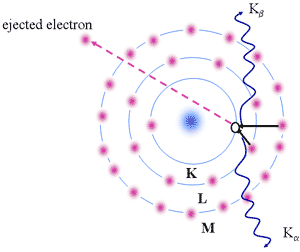### The Nucleus

• Terminology
• Binding Energies
• Nuclear Models

### Isotopes and Nuclides• A is atomic mass number (protons + neutrons)
• Z is atomic number (protons)
• N is neutron number
• X is chemical symbol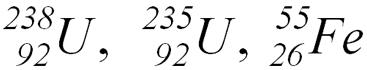• Isotopes (U)
• Nuclides (U, Fe)

### Binding Energy

Hypothetical energy released if a nuclide were synthesized from Z separate H atoms and N (equal to A - Z) separate neutrons.

Average binding energy per nucleon shows a maximum at 56Fe falling off gradually on both sides to about 7 MeV at 4He and to about 7.4 MeV for the most massive nuclei known.

Most of the naturally occurring nuclei are not stable in an absolute nuclear sense.

### Mass defect

• W = Zm(H) + (A-Z)m(N)
• weight of sum of parts > actual weight
• mass defect
• d = W  M
• represents mass equivalent of work done to separate nucleus into components,
• in energy units, called Binding energy (BE)
• BE= (W  M)amu x 931 MeV/amu
• Eb = 931(W-M)/A; MeV/nucleon

### Binding Energy per Nucleon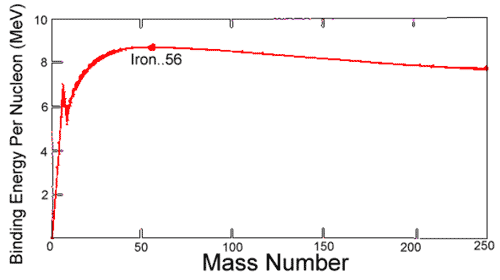### Binding Energy

• Nuclei heavier than iron gain energy by degrading into products closer to iron
• Only for heavy elements does alpha decay and spontaneous fission attain observable rates.
• Nuclear energy is gained by fusion of most elements lighter than iron
• coulombic repulsion keeps fusion rates low
• exception: if nuclei are subjected to greater than 107 K in hot cores of stars, thermonuclear bombs, controlled fusion plasmas

### Nuclear Models

• Building blocks known (n, p, subatomics)
• No definite structure defined
• Analogous to atomic system
• Three major models:
• Liquid drop
• Shell model
• Combined model

### Liquid Drop Model

Formulated (1936) by Niels Bohr and used (1939) by him and John A. Wheeler to explain nuclear fission.

Nucleons (neutrons and protons) behave like the molecules in a drop of liquid.

If given sufficient extra energy (as by the absorption of a neutron), the spherical nucleus may be distorted into a dumbbell shape and then split at the neck into two nearly equal fragments, releasing energy.

### Liquid Drop Model Bohr & Wheeler

Although inadequate to explain all nuclear phenomena, provides excellent estimates of average properties of nuclei

Key points:

• Nucleus homogenous mixture of nucleons
• Internal energy equally distributed
• Surface tension keeps nucleus spherical
• Explains nuclear fission
• Allows calculation of atomic masses
• Nuclear binding energy can be understood with the model of a charged liquid drop.

### Shell Nuclear Model

• Description of nuclei similar to Bohr atomic model of electron energy levels.
• Developed by the American physicist Robert Hofstadter in the 1950s.
• Constituent nuclear particles are paired
• neutron with neutron and proton with proton
• magic numbers: filled nuclear-energy levels when the number of protons or neutrons equals 2, 8, 20, 28, 50, 82, or 126indicate especially stable nuclei.
• The unpaired neutrons and protons account for the properties of a particular species of nucleus as valence electrons account for the chemical properties of the various elements.
• The shell model accurately predicts certain properties of normal nuclei, such as their angular momentum;

### Collective model

• Also called UNIFIED MODEL, Incorporates aspects of both the shell model and the liquid-drop model Explains certain magnetic and electric properties that neither of the two separately can explain:
• High-energy states of the nucleus
• certain magnetic and electric properties (magnetic and quadrupole moments)
• explained by the motion of the nucleons outside the closed shells combined with the motion of the paired nucleons in the core.
• Nuclear core
• thought of as a liquid drop on whose surface circulates a stable tidal bulge directed toward the rotating unpaired nucleons outside the bulge.
• Tide of protons (positively charged particles) constitutes a current that in turn contributes to the magnetic properties of the nucleus,
• the greater deformation of the nucleus as the number of unpaired nucleons increases accounts for the measured electric quadrupole moment (an index of nuclear shape -  a measure of how much the charge in space departs from spherical symmetry).

### Nuclear Decay Schemes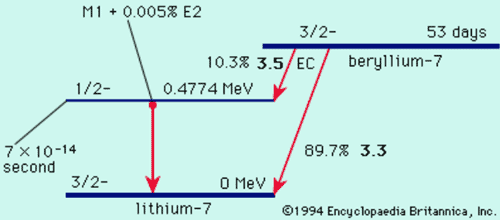### Nuclear Stability

• Binding energies
• Proton vs neutron number
• Table of isotopes

### Nuclear Stability Curve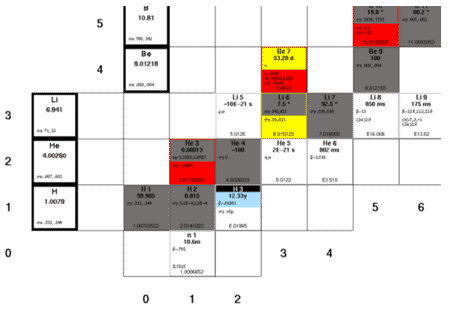Welcome Contact Getting Started Site Map Project 1 2 3 4 5 6 7 8 9 10 11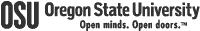College of Engineering OSU Extended Campus Local 541-737-9204 Fax 541-737-2734 4943 The Valley Library Corvallis, OR 97331-4504